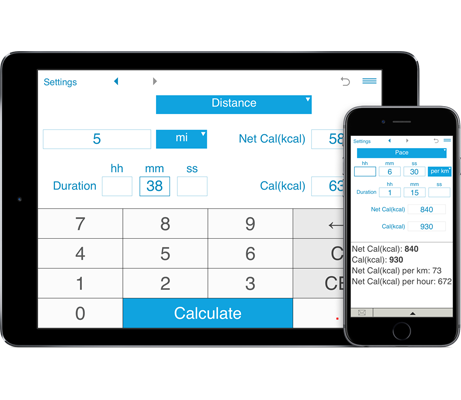# Calories Burned Running Calculator

With this calculator you can easily calculate calories burned while running, jogging and walking based on the distance / speed / pace and the duration. You can also calculate how long or what distance you should run or walk to burn the desired number of calories. The Running and Walking Calorie Calculator calculates net and gross calories you burned with running. The calculator also estimates the net calories per hour (Net Cal/hour) and net calories per mile (Net Cal/mi) or net calories per km (Net Cal/km).

## How to calculate the calories you burn while running or walking

- Net calories - calories burned with running/walking.
- Calories (or gross calories) = calories burned with running/walking + resting calories for the running/walking time.

1. Enter your personal data (age, gender, height and weight).
As the calculator makes calculations based on your age, gender, height, and weight, fill them in the "Personal" window. To get more accurate results, make sure this information is up-to-date.

2. Enter one of the following combinations:
- distance and time
- speed and time
- rhythm (pace) and time

### How to calculate distance, time, speed and pace

How long does it take to burn 200 calories by running? How far do I have to run to burn 350 calories? With the Running Calorie Calculator, you can also calculate the distance, time, speed or pace (rhythm) depending on the number of calories you want to burn.
If you have two of the parameters, you can easily calculate the third one.
- distance, time and calories
- speed, time and calories
- pace (rhythm), time and calories

#### Calories, Distance and Time

You can fill in any two fields to calculate the third value.
Enter your distance and time to know how many calories you have burned.
You can also calculate how much time or what distance you need to run to consume the desired amount of calories.

#### Calories, Speed or Pace and Time

If you know your speed or pace and the time of your workout, you can easily calculate the calories you spend while training.
Or if you know the speed / pace of your run and the number of calories you want to burn, you can easily calculate the duration.
If you know your desired number of calories and time, you can calculate how fast you should run.
Just enter any two parameters to calculate the third one.

#### How to use the Running Calorie Calculator

How to calculate burned calories based on the distance you run:
How many calories does running burn? How many calories can I burn during a 5-mile run?
It does not matter if you run a mile, 5 miles or 10 km with our running calories calculator you can estimate the amount of calories burned while running any distance at various time spans.

Enter the distance and the duration, and the calculator will show you the number of calories you burn and estimate how many calories you can burn per hour and per mile / per km.

How to calculate burned calories based on the time you run:
How many calories are burned in a 20-minute or 1-hour run?
If you know how long you run, the number of calories you burn, depends on how fast you run (you should know either the speed or the pace) and how much you weigh. The more you weigh, the more calories you burn per minute or per hour.
E.g. to calculate the number of calories you burn during a 30-minute run at 6 mph pace, select "Pace" from the drop down menu, and enter "Time" = 30 min, "Pace" = "6 mph". If you know your speed, select "Speed".